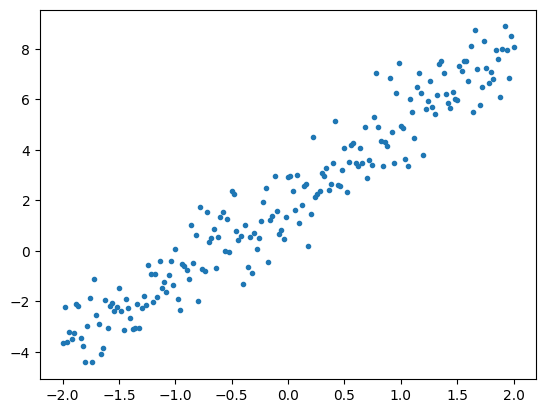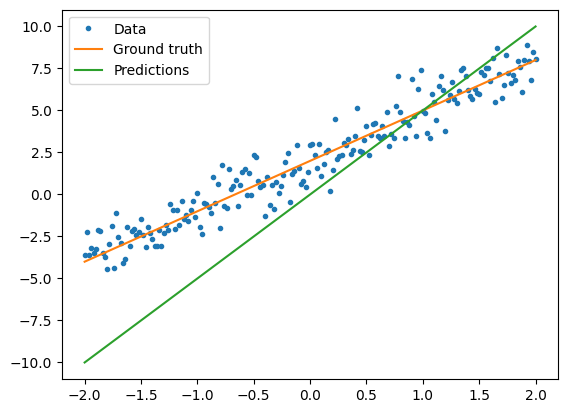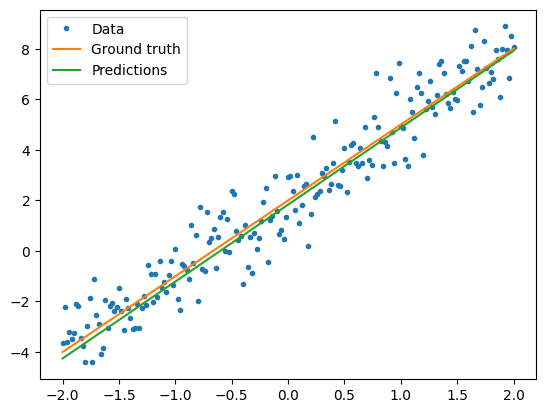# Basic training loops

In the previous guides, you have learned about tensors, variables, gradient tape, and modules. In this guide, you will fit these all together to train models.

TensorFlow also includes the tf.Keras API, a high-level neural network API that provides useful abstractions to reduce boilerplate. However, in this guide, you will use basic classes.

## Setup

import tensorflow as tf

2021-08-02 22:11:02.419697: I tensorflow/stream_executor/platform/default/dso_loader.cc:53] Successfully opened dynamic library libcudart.so.11.0


## Solving machine learning problems

Solving a machine learning problem usually consists of the following steps:

• Obtain training data.
• Define the model.
• Define a loss function.
• Run through the training data, calculating loss from the ideal value
• Calculate gradients for that loss and use an optimizer to adjust the variables to fit the data.

For illustration purposes, in this guide you'll develop a simple linear model, $f(x) = x * W + b$, which has two variables: $W$ (weights) and $b$ (bias).

This is the most basic of machine learning problems: Given $x$ and $y$, try to find the slope and offset of a line via simple linear regression.

## Data

Supervised learning uses inputs (usually denoted as x) and outputs (denoted y, often called labels). The goal is to learn from paired inputs and outputs so that you can predict the value of an output from an input.

Each input of your data, in TensorFlow, is almost always represented by a tensor, and is often a vector. In supervised training, the output (or value you'd like to predict) is also a tensor.

Here is some data synthesized by adding Gaussian (Normal) noise to points along a line.

# The actual line
TRUE_W = 3.0
TRUE_B = 2.0

NUM_EXAMPLES = 1000

# A vector of random x values
x = tf.random.normal(shape=[NUM_EXAMPLES])

# Generate some noise
noise = tf.random.normal(shape=[NUM_EXAMPLES])

# Calculate y
y = x * TRUE_W + TRUE_B + noise

2021-08-02 22:11:03.593088: I tensorflow/stream_executor/platform/default/dso_loader.cc:53] Successfully opened dynamic library libcuda.so.1
2021-08-02 22:11:04.223498: I tensorflow/stream_executor/cuda/cuda_gpu_executor.cc:937] successful NUMA node read from SysFS had negative value (-1), but there must be at least one NUMA node, so returning NUMA node zero
2021-08-02 22:11:04.224372: I tensorflow/core/common_runtime/gpu/gpu_device.cc:1733] Found device 0 with properties:
pciBusID: 0000:00:05.0 name: Tesla V100-SXM2-16GB computeCapability: 7.0
coreClock: 1.53GHz coreCount: 80 deviceMemorySize: 15.78GiB deviceMemoryBandwidth: 836.37GiB/s
2021-08-02 22:11:04.224401: I tensorflow/stream_executor/platform/default/dso_loader.cc:53] Successfully opened dynamic library libcudart.so.11.0
2021-08-02 22:11:04.227700: I tensorflow/stream_executor/platform/default/dso_loader.cc:53] Successfully opened dynamic library libcublas.so.11
2021-08-02 22:11:04.227777: I tensorflow/stream_executor/platform/default/dso_loader.cc:53] Successfully opened dynamic library libcublasLt.so.11
2021-08-02 22:11:04.228846: I tensorflow/stream_executor/platform/default/dso_loader.cc:53] Successfully opened dynamic library libcufft.so.10
2021-08-02 22:11:04.229156: I tensorflow/stream_executor/platform/default/dso_loader.cc:53] Successfully opened dynamic library libcurand.so.10
2021-08-02 22:11:04.230133: I tensorflow/stream_executor/platform/default/dso_loader.cc:53] Successfully opened dynamic library libcusolver.so.11
2021-08-02 22:11:04.231032: I tensorflow/stream_executor/platform/default/dso_loader.cc:53] Successfully opened dynamic library libcusparse.so.11
2021-08-02 22:11:04.231205: I tensorflow/stream_executor/platform/default/dso_loader.cc:53] Successfully opened dynamic library libcudnn.so.8
2021-08-02 22:11:04.231294: I tensorflow/stream_executor/cuda/cuda_gpu_executor.cc:937] successful NUMA node read from SysFS had negative value (-1), but there must be at least one NUMA node, so returning NUMA node zero
2021-08-02 22:11:04.232314: I tensorflow/stream_executor/cuda/cuda_gpu_executor.cc:937] successful NUMA node read from SysFS had negative value (-1), but there must be at least one NUMA node, so returning NUMA node zero
2021-08-02 22:11:04.233272: I tensorflow/core/common_runtime/gpu/gpu_device.cc:1871] Adding visible gpu devices: 0
2021-08-02 22:11:04.233971: I tensorflow/core/platform/cpu_feature_guard.cc:142] This TensorFlow binary is optimized with oneAPI Deep Neural Network Library (oneDNN) to use the following CPU instructions in performance-critical operations:  AVX2 AVX512F FMA
To enable them in other operations, rebuild TensorFlow with the appropriate compiler flags.
2021-08-02 22:11:04.234476: I tensorflow/stream_executor/cuda/cuda_gpu_executor.cc:937] successful NUMA node read from SysFS had negative value (-1), but there must be at least one NUMA node, so returning NUMA node zero
2021-08-02 22:11:04.235384: I tensorflow/core/common_runtime/gpu/gpu_device.cc:1733] Found device 0 with properties:
pciBusID: 0000:00:05.0 name: Tesla V100-SXM2-16GB computeCapability: 7.0
coreClock: 1.53GHz coreCount: 80 deviceMemorySize: 15.78GiB deviceMemoryBandwidth: 836.37GiB/s
2021-08-02 22:11:04.235460: I tensorflow/stream_executor/cuda/cuda_gpu_executor.cc:937] successful NUMA node read from SysFS had negative value (-1), but there must be at least one NUMA node, so returning NUMA node zero
2021-08-02 22:11:04.236440: I tensorflow/stream_executor/cuda/cuda_gpu_executor.cc:937] successful NUMA node read from SysFS had negative value (-1), but there must be at least one NUMA node, so returning NUMA node zero
2021-08-02 22:11:04.237255: I tensorflow/core/common_runtime/gpu/gpu_device.cc:1871] Adding visible gpu devices: 0
2021-08-02 22:11:04.237294: I tensorflow/stream_executor/platform/default/dso_loader.cc:53] Successfully opened dynamic library libcudart.so.11.0
2021-08-02 22:11:04.820933: I tensorflow/core/common_runtime/gpu/gpu_device.cc:1258] Device interconnect StreamExecutor with strength 1 edge matrix:
2021-08-02 22:11:04.820966: I tensorflow/core/common_runtime/gpu/gpu_device.cc:1264]      0
2021-08-02 22:11:04.820973: I tensorflow/core/common_runtime/gpu/gpu_device.cc:1277] 0:   N
2021-08-02 22:11:04.821153: I tensorflow/stream_executor/cuda/cuda_gpu_executor.cc:937] successful NUMA node read from SysFS had negative value (-1), but there must be at least one NUMA node, so returning NUMA node zero
2021-08-02 22:11:04.822099: I tensorflow/stream_executor/cuda/cuda_gpu_executor.cc:937] successful NUMA node read from SysFS had negative value (-1), but there must be at least one NUMA node, so returning NUMA node zero
2021-08-02 22:11:04.823079: I tensorflow/stream_executor/cuda/cuda_gpu_executor.cc:937] successful NUMA node read from SysFS had negative value (-1), but there must be at least one NUMA node, so returning NUMA node zero
2021-08-02 22:11:04.823889: I tensorflow/core/common_runtime/gpu/gpu_device.cc:1418] Created TensorFlow device (/job:localhost/replica:0/task:0/device:GPU:0 with 14646 MB memory) -> physical GPU (device: 0, name: Tesla V100-SXM2-16GB, pci bus id: 0000:00:05.0, compute capability: 7.0)

# Plot all the data
import matplotlib.pyplot as plt

plt.scatter(x, y, c="b")
plt.show()Tensors are usually gathered together in batches, or groups of inputs and outputs stacked together. Batching can confer some training benefits and works well with accelerators and vectorized computation. Given how small this dataset is, you can treat the entire dataset as a single batch.

## Define the model

Use tf.Variable to represent all weights in a model. A tf.Variable stores a value and provides this in tensor form as needed. See the variable guide for more details.

Use tf.Module to encapsulate the variables and the computation. You could use any Python object, but this way it can be easily saved.

Here, you define both w and b as variables.

class MyModel(tf.Module):
def __init__(self, **kwargs):
super().__init__(**kwargs)
# Initialize the weights to 5.0 and the bias to 0.0
# In practice, these should be randomly initialized
self.w = tf.Variable(5.0)
self.b = tf.Variable(0.0)

def __call__(self, x):
return self.w * x + self.b

model = MyModel()

# List the variables tf.modules's built-in variable aggregation.
print("Variables:", model.variables)

# Verify the model works
assert model(3.0).numpy() == 15.0

Variables: (<tf.Variable 'Variable:0' shape=() dtype=float32, numpy=0.0>, <tf.Variable 'Variable:0' shape=() dtype=float32, numpy=5.0>)
2021-08-02 22:11:05.452833: W tensorflow/python/util/util.cc:348] Sets are not currently considered sequences, but this may change in the future, so consider avoiding using them.


The initial variables are set here in a fixed way, but Keras comes with any of a number of initalizers you could use, with or without the rest of Keras.

### Define a loss function

A loss function measures how well the output of a model for a given input matches the target output. The goal is to minimize this difference during training. Define the standard L2 loss, also known as the "mean squared" error:

# This computes a single loss value for an entire batch
def loss(target_y, predicted_y):
return tf.reduce_mean(tf.square(target_y - predicted_y))


Before training the model, you can visualize the loss value by plotting the model's predictions in red and the training data in blue:

plt.scatter(x, y, c="b")
plt.scatter(x, model(x), c="r")
plt.show()

print("Current loss: %1.6f" % loss(y, model(x)).numpy())Current loss: 9.017786


### Define a training loop

The training loop consists of repeatedly doing three tasks in order:

• Sending a batch of inputs through the model to generate outputs
• Calculating the loss by comparing the outputs to the output (or label)
• Optimizing the variables with those gradients

For this example, you can train the model using gradient descent.

There are many variants of the gradient descent scheme that are captured in tf.keras.optimizers. But in the spirit of building from first principles, here you will implement the basic math yourself with the help of tf.GradientTape for automatic differentiation and tf.assign_sub for decrementing a value (which combines tf.assign and tf.sub):

# Given a callable model, inputs, outputs, and a learning rate...
def train(model, x, y, learning_rate):

# Trainable variables are automatically tracked by GradientTape
current_loss = loss(y, model(x))

# Use GradientTape to calculate the gradients with respect to W and b
dw, db = t.gradient(current_loss, [model.w, model.b])

# Subtract the gradient scaled by the learning rate
model.w.assign_sub(learning_rate * dw)
model.b.assign_sub(learning_rate * db)


For a look at training, you can send the same batch of x and y through the training loop, and see how W and b evolve.

model = MyModel()

# Collect the history of W-values and b-values to plot later
Ws, bs = [], []
epochs = range(10)

# Define a training loop
def training_loop(model, x, y):

for epoch in epochs:
# Update the model with the single giant batch
train(model, x, y, learning_rate=0.1)

# Track this before I update
Ws.append(model.w.numpy())
bs.append(model.b.numpy())
current_loss = loss(y, model(x))

print("Epoch %2d: W=%1.2f b=%1.2f, loss=%2.5f" %
(epoch, Ws[-1], bs[-1], current_loss))

print("Starting: W=%1.2f b=%1.2f, loss=%2.5f" %
(model.w, model.b, loss(y, model(x))))

# Do the training
training_loop(model, x, y)

# Plot it
plt.plot(epochs, Ws, "r",
epochs, bs, "b")

plt.plot([TRUE_W] * len(epochs), "r--",
[TRUE_B] * len(epochs), "b--")

plt.legend(["W", "b", "True W", "True b"])
plt.show()

Starting: W=5.00 b=0.00, loss=9.01779
Epoch  0: W=4.61 b=0.42, loss=6.11051
Epoch  1: W=4.30 b=0.75, loss=4.25310
Epoch  2: W=4.05 b=1.01, loss=3.06625
Epoch  3: W=3.85 b=1.22, loss=2.30776
Epoch  4: W=3.69 b=1.38, loss=1.82294
Epoch  5: W=3.56 b=1.52, loss=1.51299
Epoch  6: W=3.45 b=1.62, loss=1.31481
Epoch  7: W=3.37 b=1.70, loss=1.18807
Epoch  8: W=3.30 b=1.77, loss=1.10700
Epoch  9: W=3.24 b=1.82, loss=1.05513# Visualize how the trained model performs
plt.scatter(x, y, c="b")
plt.scatter(x, model(x), c="r")
plt.show()

print("Current loss: %1.6f" % loss(model(x), y).numpy())Current loss: 1.055130


## The same solution, but with Keras

It's useful to contrast the code above with the equivalent in Keras.

Defining the model looks exactly the same if you subclass tf.keras.Model. Remember that Keras models inherit ultimately from module.

class MyModelKeras(tf.keras.Model):
def __init__(self, **kwargs):
super().__init__(**kwargs)
# Initialize the weights to 5.0 and the bias to 0.0
# In practice, these should be randomly initialized
self.w = tf.Variable(5.0)
self.b = tf.Variable(0.0)

def call(self, x):
return self.w * x + self.b

keras_model = MyModelKeras()

# Reuse the training loop with a Keras model
training_loop(keras_model, x, y)

# You can also save a checkpoint using Keras's built-in support
keras_model.save_weights("my_checkpoint")

Epoch  0: W=4.61 b=0.42, loss=6.11051
Epoch  1: W=4.30 b=0.75, loss=4.25310
Epoch  2: W=4.05 b=1.01, loss=3.06625
Epoch  3: W=3.85 b=1.22, loss=2.30776
Epoch  4: W=3.69 b=1.38, loss=1.82294
Epoch  5: W=3.56 b=1.52, loss=1.51299
Epoch  6: W=3.45 b=1.62, loss=1.31481
Epoch  7: W=3.37 b=1.70, loss=1.18807
Epoch  8: W=3.30 b=1.77, loss=1.10700
Epoch  9: W=3.24 b=1.82, loss=1.05513


Rather than write new training loops each time you create a model, you can use the built-in features of Keras as a shortcut. This can be useful when you do not want to write or debug Python training loops.

If you do, you will need to use model.compile() to set the parameters, and model.fit() to train. It can be less code to use Keras implementations of L2 loss and gradient descent, again as a shortcut. Keras losses and optimizers can be used outside of these convenience functions, too, and the previous example could have used them.

keras_model = MyModelKeras()

# compile sets the training parameters
keras_model.compile(
# By default, fit() uses tf.function().  You can
# turn that off for debugging, but it is on now.
run_eagerly=False,

# Using a built-in optimizer, configuring as an object
optimizer=tf.keras.optimizers.SGD(learning_rate=0.1),

# Keras comes with built-in MSE error
# However, you could use the loss function
# defined above
loss=tf.keras.losses.mean_squared_error,
)


Keras fit expects batched data or a complete dataset as a NumPy array. NumPy arrays are chopped into batches and default to a batch size of 32.

In this case, to match the behavior of the hand-written loop, you should pass x in as a single batch of size 1000.

print(x.shape)
keras_model.fit(x, y, epochs=10, batch_size=1000)

1000
Epoch 1/10
1/1 [==============================] - 0s 185ms/step - loss: 9.0178
2021-08-02 22:11:06.212409: I tensorflow/compiler/mlir/mlir_graph_optimization_pass.cc:176] None of the MLIR Optimization Passes are enabled (registered 2)
2021-08-02 22:11:06.212965: I tensorflow/core/platform/profile_utils/cpu_utils.cc:114] CPU Frequency: 2000194999 Hz
Epoch 2/10
1/1 [==============================] - 0s 2ms/step - loss: 6.1105
Epoch 3/10
1/1 [==============================] - 0s 2ms/step - loss: 4.2531
Epoch 4/10
1/1 [==============================] - 0s 2ms/step - loss: 3.0663
Epoch 5/10
1/1 [==============================] - 0s 2ms/step - loss: 2.3078
Epoch 6/10
1/1 [==============================] - 0s 2ms/step - loss: 1.8229
Epoch 7/10
1/1 [==============================] - 0s 2ms/step - loss: 1.5130
Epoch 8/10
1/1 [==============================] - 0s 2ms/step - loss: 1.3148
Epoch 9/10
1/1 [==============================] - 0s 2ms/step - loss: 1.1881
Epoch 10/10
1/1 [==============================] - 0s 2ms/step - loss: 1.1070
<tensorflow.python.keras.callbacks.History at 0x7fe8a9dc8710>


Note that Keras prints out the loss after training, not before, so the first loss appears lower, but otherwise this shows essentially the same training performance.

## Next steps

In this guide, you have seen how to use the core classes of tensors, variables, modules, and gradient tape to build and train a model, and further how those ideas map to Keras.

This is, however, an extremely simple problem. For a more practical introduction, see Custom training walkthrough.

For more on using built-in Keras training loops, see this guide. For more on training loops and Keras, see this guide. For writing custom distributed training loops, see this guide.